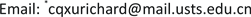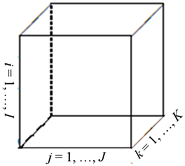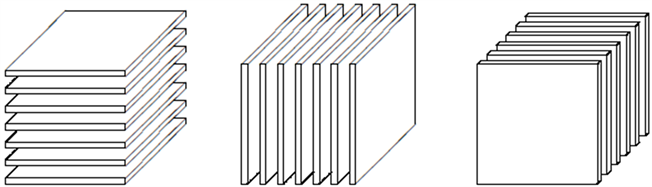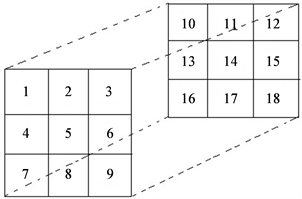﻿ 随机张量的高斯分布 On Tensor Gaussian Distributions

Vol.06 No.08(2017), Article ID:22854,8 pages
10.12677/AAM.2017.68121

On Tensor Gaussian Distributions

Lingling He, Zerong Lin, Tian Wu, Changqing Xu*

School of Mathematics and Physics, Suzhou University of Science and Technology, Suzhou Jiangsu

*通讯作者。Received: Nov. 9th, 2017; accepted: Nov. 22nd, 2017; published: Nov. 29th, 2017ABSTRACT

In this paper, we first introduce the multivariate Gaussian distributions, including the Gaussian distribution of a random vector and the Gaussian distribution of a random matrix. Some basic properties of those Gaussian distributions are also investigated. We then introduce the tensor Gaussian distribution of a random matrix, and present some basic properties for tensor Gaussian distribution.

Keywords:Tensor Gaussian Distribution, Characteristic Function, Matrix Gaussian Distribution1. 背景知识

1.1. 高斯分布的背景知识

${f}_{x}\left(x\right)=\frac{1}{\sqrt{2\text{π}{\sigma }^{2}}}\mathrm{exp}\left[-\frac{{\left(x-\mu \right)}^{2}}{2{\sigma }^{2}}\right]$ (1.1)

${f}_{z}\left(z\right)={\left(2\text{π}\right)}^{-p/2}\mathrm{exp}\left(-\frac{1}{2}{z}^{\prime }z\right)$ (1.2)

$\Sigma ={\tau }^{\prime }\tau ,\text{\hspace{0.17em}}\tau \in {R}^{p×n},\text{\hspace{0.17em}}rank\left(\tau \right)=p$ (1.3)

$x$$y=\mu +{\tau }^{\prime }z$ 具有相同分布且 $z\sim {N}_{p}\left(0,{I}_{p}\right)$ ，则称 $x\in {R}^{n}$ 服从向量高斯分布，表示为 $x\sim {N}_{n}\left(\mu ,\Sigma \right)$ 。若(1.3)中有 $n=p$ ，那么 $\Sigma$ 为正定矩阵，可得y的密度函数 

${f}_{y}\left(y\right)={\left(\text{2π}\right)}^{-n/2}{\left(\mathrm{det}\sum \right)}^{-1/2}\mathrm{exp}\left[-\frac{1}{2}{\left(y-\mu \right)}^{\prime }{\sum }^{-1}\left(y-\mu \right)\right]$ (1.4)

${Z}_{i·}\sim {N}_{m}\left(0,{I}_{m}\right),\forall i=1,2,\cdots ,m$ (1.5)

${Z}_{·j}\sim {N}_{n}\left(0,{I}_{n}\right),\forall j=1,2,\cdots ,n$ (1.6)

$\Xi ={\tau }_{1}{{\tau }^{\prime }}_{1},\text{\hspace{0.17em}}\Sigma ={\tau }_{2}{{\tau }^{\prime }}_{2},\text{\hspace{0.17em}}{\tau }_{1}\in {R}^{m×r},\text{\hspace{0.17em}}{\tau }_{2}\in {R}^{n×s}$ (1.7)

$Y$$X=\mu +{\tau }_{1}Z{{\tau }^{\prime }}_{2}$ 有相同分布，则称矩阵 $Y$ 服从矩阵高斯分布，表示为

$Y\sim {N}_{m,n}\left(\mu ,\Xi ,\Sigma \right)$ (1.8)

${f}_{Y}\left(y\right)={\left(\text{2π}\right)}^{-mn/2}{\left(\mathrm{det}\Xi \right)}^{-m/2}{\left(\mathrm{det}\Sigma \right)}^{-n/2}\mathrm{exp}\left[-\frac{1}{2}{\left(y-\mu \right)}^{\text{T}}{\Xi }^{-1}\left(y-\mu \right){\Sigma }^{-1}\right]$ (1.9)

$vec\left(Y\right)\sim {N}_{mn}\left({\mu }_{v},{\Sigma }_{v}\right)$ (1.10)

$E\left[vec\left(Y\right)\right]=E\left[vec\left(\mu \right)\right]+\left({\tau }_{2}\otimes {\tau }_{1}\right)E\left[vec\left(Z\right)\right]=vec\left(\mu \right)$

$\mathrm{cov}\left[vec\left(Y\right)\right]=\left({\tau }_{2}\otimes {\tau }_{1}\right)\mathrm{cov}\left[vec\left(Z\right)\right]{\left({\tau }_{2}\otimes {\tau }_{1}\right)}^{\prime }=\left({\tau }_{2}\otimes {\tau }_{1}\right){\left({\tau }_{2}\otimes {\tau }_{1}\right)}^{\prime }={\tau }_{2}{{\tau }^{\prime }}_{2}\otimes {\tau }_{1}{{\tau }^{\prime }}_{1}=\Phi \otimes \Xi$

1.2. 张量的背景知识Figure 1. Third order tensor $X\in {R}^{I×J×K}$

2. 预备知识

2.1. 随机向量和随机矩阵的高斯分布

(2)一个随机矩阵 $X\in {R}^{m×n}$ 的特征函数为 ${\varphi }_{X}\left(t\right)=E\left[\mathrm{exp}tr\left(i{T}^{\prime }X\right)\right]$ ，矩生成函数为 ${m}_{x}\left(t\right)=E\left[\mathrm{exp}tr\left({T}^{\prime }X\right)\right]$

(2) 若 $z\sim {N}_{m}\left(0,{I}_{m}\right)$ ，有限的常数矩阵 $\Phi \in {R}^{n×m}$ 满足 $1\le rank\left(\Phi \right)=m\le n$$y=\Phi z+\mu$ 服从向量高斯分布，记作 $y\sim {N}_{n}\left(\mu ,\Sigma \right)$ ，其中 $\Sigma =\Phi {\Phi }^{\prime }$$rank\left(\Sigma \right)=m$

(b)一个向量高斯分布 $z\sim {N}_{m}\left(\mu ,I\right)$ 有特征函数 ${\varphi }_{z}\left(t\right)={\text{e}}^{i{t}^{\prime }\mu -{t}^{\prime }t/2}$ ，矩生成函数 ${m}_{z}\left(t\right)={\text{e}}^{{t}^{\prime }\mu +{t}^{\prime }t/2}$ ，均值 $E\left(z\right)=\mu \in {\Re }^{m}$ ，协方差矩阵 $\nu \left(z\right)=I\in {\Re }^{m×m}$

(c) $y\sim {N}_{n}\left(\mu ,\Sigma \right)$ 的特征函数是 ${\varphi }_{y}\left(t\right)={\text{e}}^{i{t}^{\prime }\mu -{t}^{\prime }\Sigma t/2}$ ，矩生成函数是 ${m}_{y}\left(t\right)={\text{e}}^{{t}^{\prime }\mu +{t}^{\prime }\Sigma t/2}$

${y}_{2}\sim {N}_{{n}_{2}}\left({C}_{1}{\mu }_{1}+{c}_{0},{C}_{1}{\Sigma }_{1}{{C}^{\prime }}_{1}\right)$

${\varphi }_{Y}\left(T\right)={\varphi }_{X}\left({A}^{\prime }TB\right)\mathrm{exp}\left[itr\left({T}^{\prime }C\right)\right]$ (2.1)

$\begin{array}{c}{\varphi }_{Y}\left(T\right)=E\left[\mathrm{exp}\left\{tr\left[i{T}^{\prime }\left(AXB+C\right)\right]\right\}\right]=E\left\{\mathrm{exp}\left[tr\left(i{T}^{\prime }AXB\right)+tr\left(i{T}^{\prime }C\right)\right]\right\}\\ =E\left\{\mathrm{exp}\left[tr\left(i{\left({A}^{\prime }T{B}^{\prime }\right)}^{\prime }X\right)\right]\right\}\mathrm{exp}\left[itr\left({T}^{\prime }C\right)\right]={\varphi }_{X}\left({A}^{\prime }T{B}^{\prime }\right)\mathrm{exp}\left[itr\left({T}^{\prime }C\right)\right]\end{array}$

$\mathrm{cov}\left[co{l}_{i}\left(Y\right)\right]=\Xi ,\text{\hspace{0.17em}}\mathrm{cov}\left[vec\left(ro{w}_{i}\left(Y\right)\right)\right]=\Sigma$

(1) $vec\left(Y\right)\sim {N}_{np}\left[vec\left(M\right),\Sigma \otimes \Xi \right]$

(2) $vec\left({Y}^{\prime }\right)\sim {N}_{np}\left[vec\left({M}^{\prime }\right),\Xi \otimes \Sigma \right]$

(3) $Y=\Psi Z{\Phi }^{\prime }+M$ ，其中 $Z\sim {N}_{{n}_{1},{p}_{1}}\left(0,{I}_{{n}_{1}},{I}_{{p}_{1}}\right)$$\Xi =\Psi {\Psi }^{\prime },\text{\hspace{0.17em}}\Psi \in {R}^{n×{n}_{1}}$

$rank\left(\Psi \right)={n}_{1},\Sigma =\Phi {\Phi }^{\prime },\Phi \in {R}^{p×{p}_{1}},rank\left(\Phi \right)={p}_{1}$

$\varphi \left(T;Z\right)=E\left\{\mathrm{exp}\left[tr\left(i{T}^{\prime }Z\right)\right]\right\}=\mathrm{exp}\left[-tr\left({T}^{\prime }T\right)/2\right]$

${\varphi }_{Y}\left(T\right)=\mathrm{exp}\left[itr\left({T}^{\prime }M\right)\right]\mathrm{exp}\left[-tr\left({T}^{\prime }\Xi T\Sigma \right)/2\right]$

$Y=AXB+C\sim {N}_{n,p}\left(AMB+C,A\Xi {A}^{\prime },{B}^{\prime }\Sigma B\right)$ (2.2)

$\begin{array}{c}{\varphi }_{Y}\left(T\right)=\mathrm{exp}\left[itr\left({T}^{\prime }M\right)\right]\mathrm{exp}\left\{-tr\left[{\left({\Psi }^{\prime }T\Phi \right)}^{\prime }\left({\Psi }^{\prime }T\Phi \right)/2\right]\right\}\\ =\mathrm{exp}\left[itr\left({T}^{\prime }M\right)\right]\mathrm{exp}\left\{-tr\left({\Phi }^{\prime }{T}^{\prime }\Psi {\Psi }^{\prime }T\Phi \right)/2\right\}\\ =\mathrm{exp}\left[itr\left({T}^{\prime }M\right)\right]\mathrm{exp}\left[-tr\left({T}^{\prime }\Psi {\Psi }^{\prime }T\Phi {\Phi }^{\prime }\right)/2\right]\\ =\mathrm{exp}\left[itr\left({T}^{\prime }M\right)\right]\mathrm{exp}\left[-tr\left({T}^{\prime }\Xi T\Sigma \right)/2\right]\end{array}$

2.2. 张量的预备知识

${A}_{\left(1\right)}=\left[\begin{array}{cccccc}1& 2& 3& 10& 11& 12\\ 4& 5& 6& 13& 14& 15\\ 7& 8& 9& 16& 17& 18\end{array}\right]$ , ${A}_{\left(2\right)}=\left[\begin{array}{cccccc}1& 4& 7& 10& 13& 16\\ 2& 5& 8& 11& 14& 17\\ 3& 6& 9& 12& 15& 18\end{array}\right]$ ,

${A}_{\left(3\right)}=\left[\begin{array}{ccccccccc}1& 4& 7& 2& 5& 8& 3& 6& 9\\ 10& 13& 16& 11& 14& 17& 12& 15& 18\end{array}\right]$ .(a) horizontal切片 (b) lateral切片 (c) frontal切片

Figure 2. Slice of the third order tensor in three different directionsFigure 3. An 3 × 3 × 2 tensor A

${Y}_{{i}_{1}\cdots {i}_{n-1}j{i}_{n+1}\cdots {i}_{m}}=\underset{{i}_{n}=1}{\overset{{I}_{n}}{\sum }}{x}_{{i}_{1}\cdots {i}_{n}\cdots {i}_{m}}{u}_{j{i}_{n}}$ (2.3)

$Y=X{×}_{n}U⇔{Y}_{\left(n\right)}=U{X}_{\left(n\right)}$ (2.4)

$X{×}_{n}A{×}_{m}B=X{×}_{m}B{×}_{n}A$ (2.5)

3. 随机张量的高斯分布

$Α\sim {N}_{m,n,p}\left(Μ,{\Sigma }_{1},{\Sigma }_{2},{\Sigma }_{3}\right)$ (3.1)

${Α}_{\left(1\right)j}\sim {N}_{m}\left({Μ}_{\left(1\right)j},{\Sigma }_{1}\right)$ , ${Α}_{\left(2\right)j}\sim {N}_{n}\left({Μ}_{\left(2\right)j},{\Sigma }_{2}\right)$ , ${Α}_{\left(3\right)j}\sim {N}_{p}\left({Μ}_{\left(3\right)j},{\Sigma }_{3}\right)$

${Α}_{\left(1\right)}\sim {N}_{m,np}\left({Μ}_{\left(1\right)},{\Sigma }_{1},{\Sigma }_{3}\otimes {\Sigma }_{2}\right)$ (3.2)

${Α}_{\left(2\right)}\sim {N}_{n,mp}\left({Μ}_{\left(2\right)},{\Sigma }_{2},{\Sigma }_{3}\otimes {\Sigma }_{1}\right)$ (3.3)

${Α}_{\left(3\right)}\sim {N}_{p,mn}\left({Μ}_{\left(3\right)},{\Sigma }_{3},{\Sigma }_{2}\otimes {\Sigma }_{1}\right)$ (3.4)

$vec\left({B}^{\prime }\right)\sim {N}_{mp}\left[vec\left(Μ\left(j,:,:\right)\right),{\Sigma }_{3}\otimes {\Sigma }_{2}\right]$$vec\left(C\right)\sim {N}_{mp}\left[vec\left(Μ\left(:,j,:\right)\right),{\Sigma }_{3}\otimes {\Sigma }_{1}\right]$

$vec\left(D\right)\sim {N}_{mp}\left[vec\left(Μ\left(:,:,j\right)\right),{\Sigma }_{2}\otimes {\Sigma }_{1}\right]$

${Α}_{\left(1\right)}\sim {N}_{m,np}\left({Μ}_{\left(1\right)},{\Sigma }_{1},{\Sigma }_{3}\otimes {\Sigma }_{2}\right)$${Α}_{\left(2\right)}\sim {N}_{n,mp}\left({Μ}_{\left(2\right)},{\Sigma }_{2},{\Sigma }_{3}\otimes {\Sigma }_{1}\right)$${Α}_{\left(3\right)}\sim {N}_{p,mn}\left({Μ}_{\left(3\right)},{\Sigma }_{3},{\Sigma }_{2}\otimes {\Sigma }_{1}\right)$

(1) $vec\left({Α}_{\left(1\right)}\right)\sim {N}_{mnp}\left(vec\left({Μ}_{\left(1\right)}\right),{\Sigma }_{3}\otimes {\Sigma }_{2}\otimes {\Sigma }_{1}\right)$

(2) $vec\left({Α}_{\left(2\right)}\right)\sim {N}_{nmp}\left(vec\left({Μ}_{\left(2\right)}\right),{\Sigma }_{3}\otimes {\Sigma }_{1}\otimes {\Sigma }_{2}\right)$

(3) $vec\left({Α}_{\left(3\right)}\right)\sim {N}_{pmn}\left(vec\left({Μ}_{\left(3\right)}\right),{\Sigma }_{2}\otimes {\Sigma }_{1}\otimes {\Sigma }_{3}\right)$

$Β=Α{×}_{1}{T}_{1}{×}_{2}{T}_{2}{×}_{3}{T}_{3}+U\sim {N}_{m,n,p}\left(Α{×}_{1}{T}_{1}{×}_{2}{T}_{2}{×}_{3}T+U,{T}_{1}{\Sigma }_{1}{{T}^{\prime }}_{1},{T}_{2}{\Sigma }_{2}{{T}^{\prime }}_{2},{T}_{3}{\Sigma }_{3}{{T}^{\prime }}_{3}\right)$ (3.5)

$\Lambda =Α{×}_{2}{T}_{2}{×}_{3}{T}_{3}$ ，则 ${\Lambda }_{\left(1\right)}={Α}_{\left(1\right)}{\left({T}_{3}\otimes {T}_{2}\right)}^{\prime }$ ，则 ${Β}_{\left(1\right)}={T}_{1}{Α}_{\left(1\right)}{\left({T}_{3}\otimes {T}_{2}\right)}^{\prime }+{U}_{\left(1\right)}$

${Β}_{\left(1\right)}={T}_{1}{Α}_{\left(1\right)}{\left({T}_{3}\otimes {T}_{2}\right)}^{\prime }+{U}_{\left(1\right)}\sim {N}_{m,np}\left({T}_{1}{M}_{\left(1\right)}{\left({T}_{3}\otimes {T}_{2}\right)}^{\prime }+{U}_{\left(1\right)},{T}_{1}{\Sigma }_{1}{{T}^{\prime }}_{1},\left({T}_{3}\otimes {T}_{2}\right)\left({\Sigma }_{3}\otimes {\Sigma }_{2}\right){\left({T}_{3}\otimes {T}_{2}\right)}^{\prime }\right)$

$\left({T}_{3}\otimes {T}_{2}\right)\left({\Sigma }_{3}\otimes {\Sigma }_{2}\right){\left({T}_{3}\otimes {T}_{2}\right)}^{\prime }=\left({T}_{3}{\Sigma }_{3}{{T}^{\prime }}_{3}\right)\otimes \left({T}_{2}{\Sigma }_{2}{{T}^{\prime }}_{2}\right)$

$Β=Α{×}_{1}{T}_{1}{×}_{2}{T}_{2}{×}_{3}{T}_{3}+U\sim {N}_{m,n,p}\left(Α{×}_{1}{T}_{1}{×}_{2}{T}_{2}{×}_{3}T+U,{T}_{1}{\Sigma }_{1}{{T}^{\prime }}_{1},{T}_{2}{\Sigma }_{2}{{T}^{\prime }}_{2},{T}_{3}{\Sigma }_{3}{{T}^{\prime }}_{3}\right)$

On Tensor Gaussian Distributions[J]. 应用数学进展, 2017, 06(08): 1010-1017. http://dx.doi.org/10.12677/AAM.2017.68121

1. 1. Chikuse, Y. (2008) The Matrix Angular Central Gaussian Distribution. Journal of Multivariate Analysis, 33, 265-274.
https://doi.org/10.1016/0047-259X(90)90050-R

2. 2. 尉迟江. 对高斯分布函数形式的推导[J]. 统计与信息论坛, 2009, 24(5): 3-6.

3. 3. 熊焰, 赵铁山. 金融资产收益率分布的混合高斯分布模型[J]. 统计与决策, 2004(12): 26.

4. 4. 张乃根. 经济学分析法学的理论基石——“高斯原理”评介[J]. 政治与法律, 1991(2): 54-56.

5. 5. 邓改革. 天文时间序列的分析研究[D]: [硕士学位论文]. 广州: 广州大学, 2013.

6. 6. 徐甲文, 徐松涛. 指数或高斯分布信号的MSE量化器的简捷设计[J]. 北京航空航天大学学报, 1997(3): 404-408.

7. 7. 李建泉. 正态分布高斯推证法释疑[J]. 测绘地理信息, 1991(1): 35-37.

8. 8. 王海涌, 费峥红, 王新龙. 基于高斯分布的星像点精确模拟及质心计算[J]. 光学精密工程, 2009, 17(7): 1672-1677.

9. 9. Kollo, T. and Von Rosen, D. (2005) Advanced Multivariate Statistics with Matrices. Springer Netherlands, 81-82, 123-135.

10. 10. Muller, K.E. and Stewart, P.W. (2006) Linear Model Theory. Wiley-Interscience [John Wiley & Sons], Hoboken, NJ, 7-8.
https://doi.org/10.1002/0470052147

11. 11. Kolda, T.G. and Badel, B.W. (2009) Tensor Decompositions and Ap-plications. Sandia Report, 51, 455-500.
https://doi.org/10.1137/07070111X

12. 12. Qi, L. and Luo, Z. (2017) Tensor Analysis: Spectral Theory and Spectral Tensors. SIAM.
https://doi.org/10.1137/1.9781611974751